## Friday, October 26, 2007

I've recently gone to Northwest Probability Seminar and one topic that came up was Thorp shuffling:

Take a deck of cards, split it in 2 evenly, then proceed to riffle them by dropping card from stack x, followed by card from the other stack, where x could be stack 1 or stack 2 with equal probability. So if we have 4 cards, numbered 1..4, then 1234 will become one of 1324,1342,3124,2142 after one shuffle. How many times should you shuffle until the deck is randomized?

Solving this for n cards has been called the "longest standing open problem in the theory of mixing times".

Since card shuffling is a random walk on a vertex transitive graph, so we could check what that graph looks like.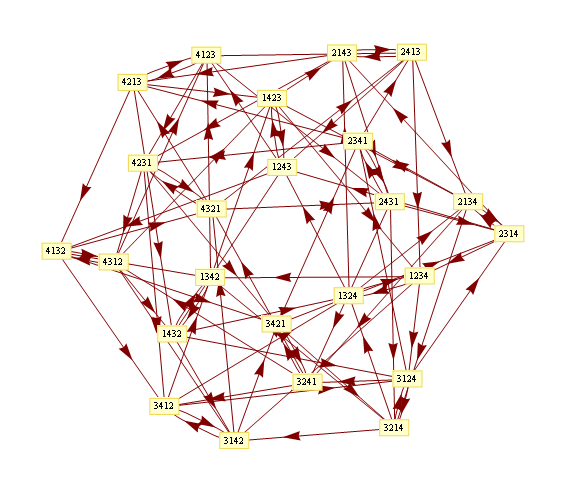Mathematica's graph drawing algorithms doesn't take into account special vertex transitive structure, so the graph will looks like a mess.

Going to 3D doesn't help much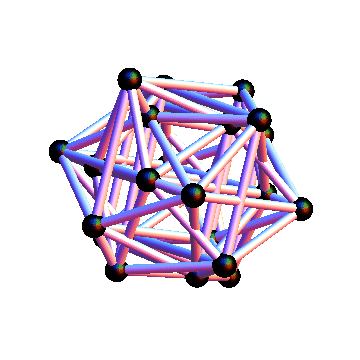One way to discover structure of a matrix is to decompose it into a sum of rank 1 matrices.

Here's the adjacency matrix of the graph above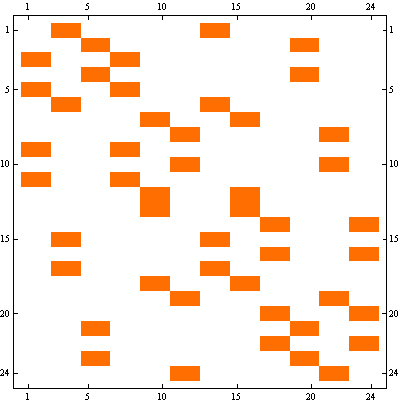We can represent it as a sum of following 6 rank-1 matrices.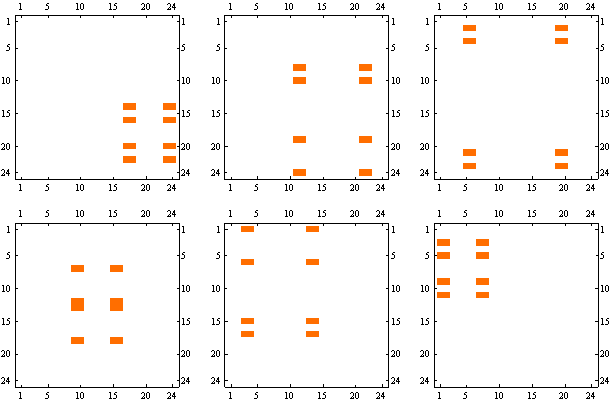We can look at the graph corresponding to each of these matrices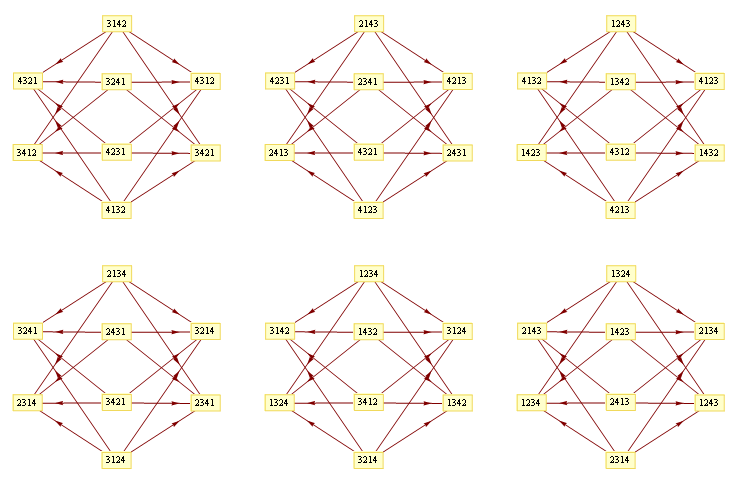This shows all the transitions present in the original graph, but you can see much more structure. In particular, all 6 graphs are isomorphic and bipartite, and the arrows are going only one way between partitions. If we put nodes in each "source" partition into one node, you can see an overall structure of the graph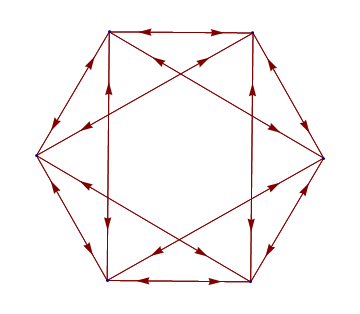Finally, we can arrange the nodes in the original graph so that the nodes in the same partition are close together, and here's the result: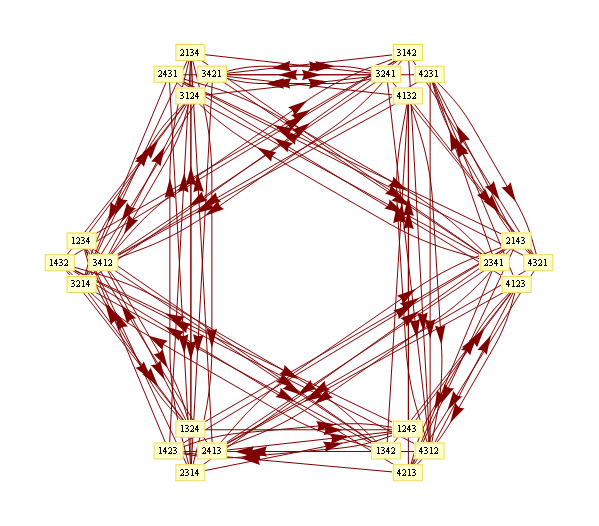Here's the Mathematica notebook I used to produce these figures. Web versionAnonymous said...

I'd be curious whether that very last graph could be arranged in some interesting way in 3D. Like if you put the decomposed graphs on adjacent planes and then just connected them, maybe. It ALMOST even looks like, if you're careful, you might even be able to map the subgraphs to the faces of some polyhedron-like object or something...

Yaroslav Bulatov said...

Good point, a quick check shows that this maps to an octahedron. You can see the overall structure looks the same as the second embedding here http://mathworld.wolfram.com/OctahedralGraph.html

draj said...

Excellent machine learning blog,thanks for sharing...
Seo Internship in Bangalore
Smo Internship in Bangalore
Digital Marketing Internship Program in Bangalore

john said...
john said...